## Extremal Graph

A two-coloring of a Complete Graph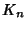ofnodes which contains exactly the number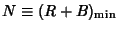of Monochromatic Forced Triangles and no more (i.e., a minimum of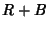where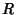and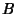are the numbers of red and blue Triangles). Goodman (1959) showed that for an extremal graph,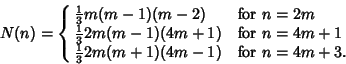This is sometimes known as Goodman's Formula. Schwenk (1972) rewrote it in the form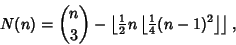sometimes known as Schwenk's Formula, where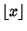is the Floor Function. The first few values of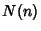for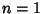, 2, ... are 0, 0, 0, 0, 0, 2, 4, 8, 12, 20, 28, 40, 52, 70, 88, ... (Sloane's A014557).

See also Bichromatic Graph, Blue-Empty Graph, Goodman's Formula, Monochromatic Forced Triangle, Schwenk's Formula

References

Goodman, A. W. On Sets of Acquaintances and Strangers at Any Party.'' Amer. Math. Monthly 66, 778-783, 1959.

Schwenk, A. J. Acquaintance Party Problem.'' Amer. Math. Monthly 79, 1113-1117, 1972.

Sloane, N. J. A. Sequence A014557 in The On-Line Version of the Encyclopedia of Integer Sequences.'' http://www.research.att.com/~njas/sequences/eisonline.html.